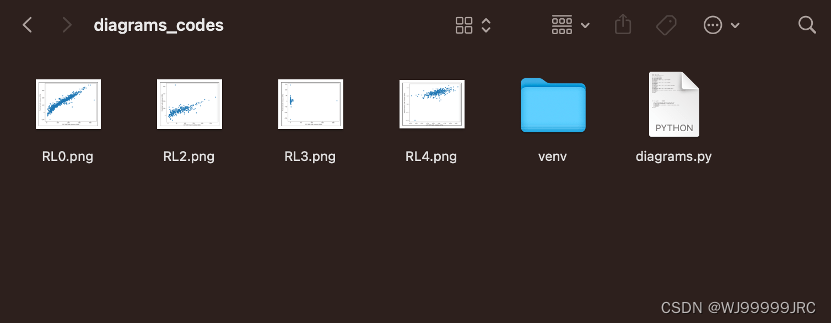# 使用Python中的matplotlib将多个图片显示到一张图内``````import matplotlib.pyplot as plt

``````plt.subplot(221, xlabel = '(1)', title = "K_VRH")
plt.imshow(im)``````

`前文提到的plt.imread`函数通常与其他 Matplotlib 库中的函数一起使用，例如 `plt.imshow`，用于显示和处理图像数据。

``````plt.xticks([])
plt.yticks([])``````

``````plt.subplot(222, xlabel = '(2)', title = "G_VRH")
plt.imshow(im2)
plt.xticks([])
plt.yticks([])
plt.subplot(223, xlabel = '(3)', title = "Elastic anisotropy")
plt.imshow(im3)
plt.xticks([])
plt.yticks([])
plt.subplot(224, xlabel = '(4)', title = "Poisson ratio")
plt.imshow(im4)
plt.xticks([])
plt.yticks([])``````

``````plt.subplots_adjust(left=None, bottom=None, right=None, top=None, wspace=None, hspace=0.5)
plt.show()``````